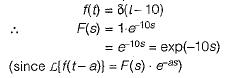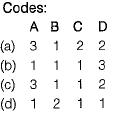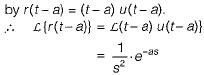Courses

# Introduction

## 10 Questions MCQ Test Topicwise Question Bank for GATE Electrical Engineering | Introduction

Description
This mock test of Introduction for Electronics and Communication Engineering (ECE) helps you for every Electronics and Communication Engineering (ECE) entrance exam. This contains 10 Multiple Choice Questions for Electronics and Communication Engineering (ECE) Introduction (mcq) to study with solutions a complete question bank. The solved questions answers in this Introduction quiz give you a good mix of easy questions and tough questions. Electronics and Communication Engineering (ECE) students definitely take this Introduction exercise for a better result in the exam. You can find other Introduction extra questions, long questions & short questions for Electronics and Communication Engineering (ECE) on EduRev as well by searching above.
QUESTION: 1

### The Lapiace transform of a transportation Iag of 10 seconds is

Solution:QUESTION: 2

Solution:
QUESTION: 3

### Linear system obeys

Solution:
QUESTION: 4

The most commonly used input signal(s) in control system is/are

Solution:
QUESTION: 5

Consider the following statements regarding control systems:
1. In open loop control system, the control action depends upon the desired output.
2. All control systems operated by present timing mechanism are open loop.
3. in a closed loop control system feedback signal is usually negative.
4. In open-loop control system stability cannot be ensured.

Q. Which of the above statements are correct?

Solution:
• There is no-control action in open loop systems. Hence, statement 1 is false.
• Generally, all control systems operated by present timing mechanism are open loop because they are more stable than closed loop control system. Hence, statement-2 is true.
• Due to various advantages of negative feedback systems (except reduction in gain), feedback signal is usually negative in a closed loop control system. Hence, statement-3 is true.
• In open-loop control systems stability can be ensured because they are more stable than closed-loop control system.
• Thus, only statements 2 and 3 are true.
QUESTION: 6

Assertion (A): Closed loop control systems are known as feedback control systems.
Reason (R): In closed loop control systems, the control action is dependent on the desired output.

Solution:
QUESTION: 7

As compared to a closed loop system, an open loop system is

Solution:

The main difference between an open-loop system and a closed-loop system is that the closed-loop system has the ability to self-correct while the open-loop system doesn't. Consequently, closed-loop systems are often called feedback control systems while open-loop systems are also known as non-feedback controls.

QUESTION: 8

Match List-I with List-ll and select the correct answer using the codes given below the lists:
List-I
A. Immersion rod
B. Air conditioner
C. Automatic washing machine
D. Automatic control of traffic
List-ll
1. Open loop control system
2. Closed loop control system
3. Neither a closed loop nor an open loop systemSolution:
QUESTION: 9

Assertion (A): The control system which operates on the time basis is an open loop system.
Reason (R): A field control d.c. motor is an example of open loop system.

Solution:

The control system which operates on the time basis is an open loop system. For example, in automatic control of traffic, the time for each lamp (red, yellow and green) is fixed. The operation of each lamp does not depends upon the density of the traffic but depends upon the fixed time. Hence, assertion is true.
Reason is also true but, it is not the correct explanation of assertion.

QUESTION: 10

A ramp function occurs at t = a. It's Laplace transform is

Solution:

Ramp function occurring at t = 0 is denoted by r(t) = tu(t).
Ramp function occurring at t - a will be denoted